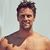# You're Brilliant If You Smash These Algebra Equations

## Do you have the traits of highly intelligent people? Test your math knowledge with this super hard quiz.Created by Michael Rogers
On Aug 29, 2021
1 / 15

25 ÷ 5 (10 − 5) = ?

2 / 15

1 + 1 × ¼ + 1 − 1 = ?

3 / 15

7 + 1 x 1 + 8 ÷ 2 = ?

4 / 15

5 + 3 (5 + 3) = ?

5 / 15

8 + 8 ÷ 8 + 8 x 8 – 8 = ?

6 / 15

4 + 1 × 4 + 4 − 1 = ?

7 / 15

5 + 1 x 0 + 8 ÷ 2 = ?

8 / 15

20 – 20 ÷ ¼ + 1 = ?

9 / 15

8 ÷ 4 (4 – 2) = ?

10 / 15

20 – 4 ÷ ¼ + 8 = ?

11 / 15

20 ÷ 4 x 10 ÷ 2 = ?

12 / 15

6 ÷ 2 (2 + 1) = ?

13 / 15

8 ÷ 2 + 4 (8 − 4) = ?

14 / 15

8 ÷ 4 (4 – 2) = ?

15 / 15

6 – 1 x 0 + 3 ÷ 3 = ?

15
Questions left
These are 10 of the World CRAZIEST Ice Cream Flavors
Created by Tal Garner
On Nov 18, 2021